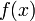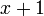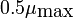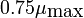# FAQ: Why does the effective service rate f(x) go to zero when x = 0 in Example 2.10 on queuing systems?

Jump to: navigation, search

(Contributed by Richard Murray, 10 Oct 09)

Q: The formula forwhich scalesis. This is zero when x = 0 (no queue) and 1 when x goes to infinity. Why is this the right model?

A: The model used by Agnew  is that the rate at which jobs are processed is linear in the queue length when the length is small, and saturates and the maximum service rate. At the extreme where there are no jobs on the queue, there is no need to process incoming requests and Agnew's assumption was the more processing would be applied as the queue got longer, until it saturates forvery large.

Notice that the termin the denominator means that the service rate very rapidly reaches it maximum as the queue increases. If there is 1 job on the queue then the service rate is, 3 jobs gives a rate ofand 9 jobs gives a rate of.